# Trace of a square matrix

(diff) ← Older revision | Latest revision (diff) | Newer revision → (diff)

The sum of the entries on the main diagonal of this matrix. The trace of a matrix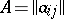is denoted by,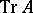or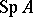: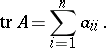Letbe a square matrix of orderover a field. The trace ofcoincides with the sum of the roots of the characteristic polynomial of. Ifis a field of characteristic 0, then thetraces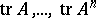uniquely determine the characteristic polynomial of. In particular,is nilpotent if and only if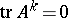for all.

Ifandare square matrices of the same order over, and, then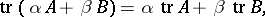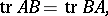while if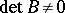,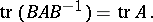The trace of the tensor (Kronecker) product of square matrices over a field is equal to the product of the traces of the factors.